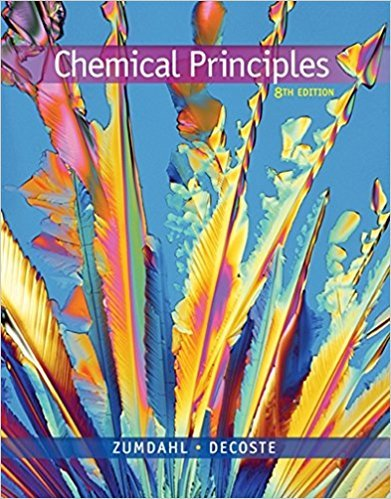×
×

# Portland cement acts as the binding agent in concrete. AISBN: 9781305581982 176

## Solution for problem 3.54 Chapter 3

Chemical Principles | 8th Edition

• Textbook Solutions
• 2901 Step-by-step solutions solved by professors and subject experts
• Get 24/7 help from StudySoup virtual teaching assistantsChemical Principles | 8th Edition

4 5 0 296 Reviews
11
4
Problem 3.54

Portland cement acts as the binding agent in concrete. A typical Portland cement has the following composition: Formula Name Mass Percent Ca3SiO5 Tricalcium silicate 50. Ca2SiO4 Dicalcium silicate 25 Ca3Al2O6 Tricalcium aluminate 12 Ca2AlFeO5 Calcium aluminoferrite 8.0 CaSO4 ? 2H2O Calcium sulfate dihydrate 3.5 Other substances, mostly MgO 1.5 Assuming that the impurities contain no Ca, Al, or Fe, calculate the mass percent of these elements in this Portland cement.

Step-by-Step Solution:
Step 1 of 3

Chase Bobier CHEM 1010 notes week 8 Important Terms Things you need to know Equations and Calculations The mole (abbreviated mol) is a way of being able to accurately know how many atoms of an element or molecules of a compound are contained in a sample. A mole is 6.02x10 23items, and is an elements atomic weight in grams. 1 mol Zing = 6.02x10 23Zinc...

Step 2 of 3

Step 3 of 3

##### ISBN: 9781305581982

This textbook survival guide was created for the textbook: Chemical Principles, edition: 8. This full solution covers the following key subjects: . This expansive textbook survival guide covers 20 chapters, and 2754 solutions. The answer to “Portland cement acts as the binding agent in concrete. A typical Portland cement has the following composition: Formula Name Mass Percent Ca3SiO5 Tricalcium silicate 50. Ca2SiO4 Dicalcium silicate 25 Ca3Al2O6 Tricalcium aluminate 12 Ca2AlFeO5 Calcium aluminoferrite 8.0 CaSO4 ? 2H2O Calcium sulfate dihydrate 3.5 Other substances, mostly MgO 1.5 Assuming that the impurities contain no Ca, Al, or Fe, calculate the mass percent of these elements in this Portland cement.” is broken down into a number of easy to follow steps, and 70 words. Since the solution to 3.54 from 3 chapter was answered, more than 227 students have viewed the full step-by-step answer. Chemical Principles was written by and is associated to the ISBN: 9781305581982. The full step-by-step solution to problem: 3.54 from chapter: 3 was answered by , our top Chemistry solution expert on 12/23/17, 04:53PM.

Unlock Textbook Solution